# How to Sum If Date is Greater or Less Than in Excel

If we sum for certain conditions, we’ll use the SUMIF function in excel. For example, count the total sales for a period before of after a specified date. This article will help you to do sum for a specified period by SUMIF function.

## SUMIF Date is Less/Greater than Specified Date in Excel

Prepare a table with dates and sales.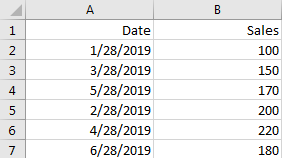If we want to count the total sales for dates before a specified date, we can follow below steps to do this.

Step 1: Prepare another table to save the specified date and total sales.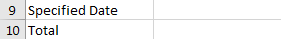Step 2: type the Specified Date in Cell. For example: 5/28/2019; then enter the below formula based on the SUMIF function:

`=SUMIF(A2:A7,"<"&B9,B2:B7)`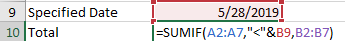In above formula, A2:A7 is the range of all dates, B9 is the specified date, B2:B7 is the range of sales. You can change the date and range in the formula based on your needs.

Step 3: Click Enter to get the result.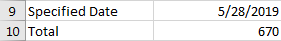If you want to count the total for sales greater than a specified date and includes that date, you can use below formula.

Step 4: In B10, enter the below formula:

`=SUMIF(A2:A7,">="&B9,B2:B7)`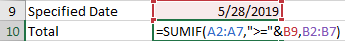Step 5: Click Enter to get the result.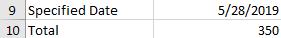Note:

If you want count the total sales before today, you can directly enter the following formula based on the SUMIF function and the TODAY function:

`=SUMIF(A2:A7,"<"&TODAY(),B2:B7)`

### Related Functions

• Excel TODAY function
The Excel TODAY function returns the serial number of the current date. So you can get the current system date from the TODAY function. The syntax of the TODAY function is as below:=TODAY()…
• Excel SUMIF Function
The Excel SUMIF function sum the numbers in the range of cells that meet a single criteria that you specify. The syntax of the SUMIF function is as below:=SUMIF (range, criteria, [sum_range])…

Sidebar